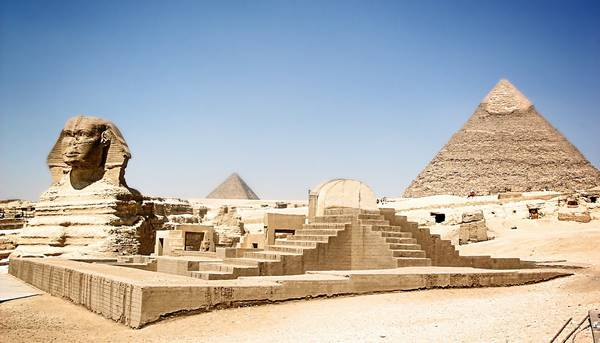# 金字塔一组数字蕴含奇特规律 至今无解“142857” 这组数字据称是从埃及金字塔里面发现的，乍看起来没有什么特别，稍微细看会发现这组数字不包含3、6、9，更加仔细研究后，人们发现了一些匪夷所思的奇特规律。

142857 × 1 = 14285(7) 1*7=7
142857 × 2 = 28571(4) 2*7=14
142857 × 3 = 42857(1) 3*7=21
142857 × 4 = 57142(8) 4*7=28
142857 × 5 = 71428(5) 5*7=35
142857 × 6 = 85714(2) 6*7=42
142857 × 7 = 99999(9) 7*7=49
142857 × 8 = 114285(6) 8*7=56
142857 × 9 = 128571(3) 9*7=63
142857 × 10 = 142857(0) 10*7=70

20408+122449=142857这组数字又回到了最初的模样。

Copyright © 2007 - 2020 , Design by 真相网. 本站资料可以免费自由转载. 若有版权问题请留言通知本站管理员. 【回到顶部】